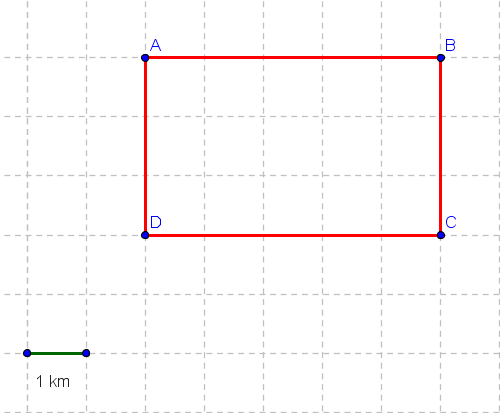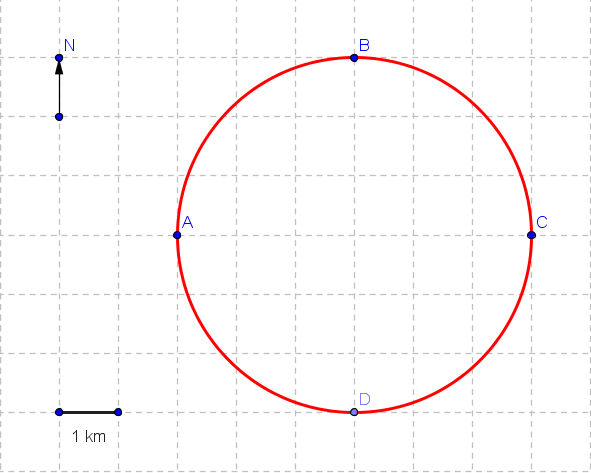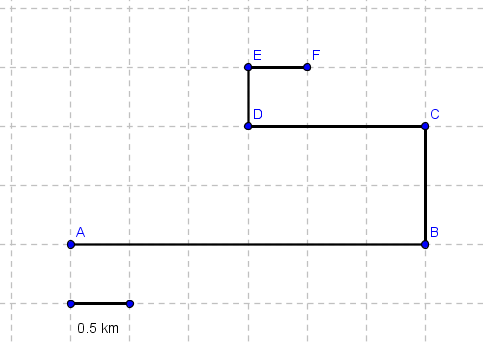# Displacement and Distance: Problems with Solutions

Problems with detailed solutions on displacement and distance of moving objects.

### Problem 1

An object moves from point A to point B to point C, then back to point B and then to point C along the line shown in the figure below.
a) Find the distance covered by the moving object.
b) Find the magnitude and direction of the displacement of the object.Solution to Problem 1

### Problem 2

An object moves from point A to point C along the rectangle shown in the figure below.
a) Find the distance covered by the moving object.
b) Find the magnitude of the displacement of the object.Solution to Problem 2

Problem 3: An object moves from point A to B to C to D and finally to A along the circle shown in the figure below.
a) Find the distance covered by the moving object.
b) Find the magnitude and direction of the displacement of the object.Solution to Problem 3

### Problem 4

An object moves from point A to B to C to D along the circle shown in the figure below.
a) Find the distance covered by the moving object.
b) Find the magnitude of the displacement of the object.Solution to Problem 4

### Problem 5

An object moves along the grid through the points A, B, C, D, E, and F as shown below.
a) Find the distance covered by the moving object.
b) Find the magnitude of the displacement of the object.Solution to Problem 5